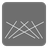Reversed Permutation Index
Simple
English

This mission is the inverse function of Permutation Index . (The mission idea is by przemyslaw.daniel .)

You are given the length of the permutation (an integer) and the permutation index (an integer starting from 1). The task is to calculate one of the permutations of the consecutive integers (starting from 0) and return it (an iterable of integers).

For example

• Input: 3, 4
• All consecutive permutations are:
1. (0, 1, 2)
2. (0, 2, 1)
3. (1, 0, 2)
4. (1, 2, 0) !!!
5. (2, 0, 1)
6. (2, 1, 0)
• Output: (1, 2, 0)

Example:

```assert tuple(reversed_permutation_index(3, 5)) == (2, 0, 1)
assert tuple(reversed_permutation_index(6, 271)) == (2, 1, 3, 0, 4, 5)
```

Input: The length of the permutation (an integer) and the permutation index (an integer).

Output: One of the permutations (an iterable of integers).

Precondition:

• 1 ≤ length
• 1 ≤ permutation_index ≤ math.factorial(length)
Settings
Code:
CheckiO Extensions

CheckiO Extensions allow you to use local files to solve missions. More info in a blog post.

In order to install CheckiO client you'll need installed Python (version at least 3.8)

Install CheckiO Client first:

`pip3 install checkio_client`

`checkio --domain=py config --key=`

Sync solutions into your local folder

`checkio sync`

(in beta testing) Launch local server so your browser can use it and sync solution between local file end extension on the fly. (doesn't work for safari)

`checkio serv -d`

Alternatevly, you can install Chrome extension or FF addon

`checkio install-plugin`
`checkio install-plugin --ff`
`checkio install-plugin --chromium`

Read more here about other functionality that the checkio client provides. Feel free to submit an issue in case of any difficulties.

Sync...